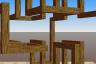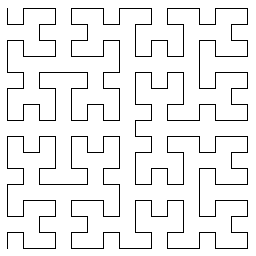http://people.csail.mit.edu/jaffer/Geometry/HSFC

# Hilbert Space-Filling Curves

A space-filling curve is a parameterized, injective function which maps a unit line segment to a continuous curve in the unit square, cube, hypercube, etc, which gets arbitrarily close to a given point in the unit cube as the parameter increases.

Space-filling curves serve as a counterexample to less-than-rigorous notions of dimension. In addition to their mathematical importance, space-filling curves have applications to dimension reduction, mathematical programming [Butz 1968], sparse multi-dimensional database indexing [Lawder 2000], and electronics [Zhu 2003].

In 2003 I found three algorithms for the n-dimensional Hilbert Space-Filling Curve (fourth found recently):

There is a surfeit of 2-dimensional space-filling curves, but generalizing them to higher rank is not necessarily practical or even possible.

I had some issues with these sources:

• All are cryptic. Butz introduces seven layers of subscripted variables to describe his algorithm in terms of boolean exclusive-or; obscuring the higher level operations (such as gray-code->integer) being done.

The C code in the Utah Raster Toolkit which implements Butz's algorithm does its processing through the use of computed tables.

The algorithm presented here is in terms of higher level constructs: Gray-code, lamination, rotation and reflection.

• The conversion functions for all take an argument for the width (in bits) of the multidimensional indexes. Although the origin of the multidimensional spaces are 0, the coordinates returned for other scalars depend on the width argument.

The algorithmic extension presented here is a bijection between N and Nn, imposing no limitations on integer size. Some datasets will no longer need to be scaled.

• The scalar must have rank times the precision of each multidimensional coordinate. C's integer size limitations spoil the algorithm for high dimensional spaces.

The algorithms presented here do not depend on, and are not bound by integer size limitations.### Self-Similarity

The Z-curve, one of the simplest space-filling functions, forms each vector coordinate from every n-th bit of the scalar. It is self-avoiding, but contains some long edges.

The transforms we are examining are self-similar. For the better behaved members of this class the vector displacements from changing a digit in the scalar are less than or equal to the vector displacements from changing a higher order digit.

### Gray codeThe simplest optimal space-filling curve is one which uses two positions on each axis.

A Gray code is an ordering of non-negative integers in which exactly one bit differs between each pair of successive elements. There are multiple Gray codings. An n-bit Gray code corresponds to a Hamiltonian cycle on an n-dimensional hypercube.Combining the Gray codes with self-similarity, we want the top-level displacements to be a Gray-coded n-dimensional hypercube. To add a layer of low-order bits, replace each stroke of the lowest-order Gray-code with a Gray-coded hypercube.

That doesn't look right! The net displacement from each hypercube is one edge. We must rotate each small hypercube so that its net displacement matches the edge it is replacing. This series of rotations starts with the most significant hypercube.

Permutations in the n bits encoding one hypercube correspond to its rotations. When n is 2, there are only two possible rotations; but as n increases, the number of distinct rotations grows to n! (n factorial).

When there are choices in which rotation to use, the resulting curves may be different. Alber and Niedermeier [Alber 1998] reveal:

..., whereas there is basically one Hilbert curve in the 2D world, our analysis shows that there are 1536 structurally different 3D Hilbert curves.It turns out that cyclic rotations (shifts which loop around) of the field of bits encoding a hypercube is sufficient for space-filling curves. We must also potentially reverse the net direction of the small hypercube to match the direction of the edge it is replacing. This is a simple matter of flipping that bit which encodes the net direction.

### The Butz Algorithm

So far this is the algorithm disclosed by [Butz 1971] and cited by Thomas and Moore. Teasing an understanding in terms of Gray-codes, lamination, rotations and reflections from the C code and Butz's papers has been quite a challenge.The Butz algorithm and its derivatives take the number of bits per coordinate as a parameter. Although the origin of the multidimensional spaces are at (0, ..., 0), the coordinates returned for other indexes depend on the width argument.

These algorithms treat their numbers as base-2 fractions; the curves generated have the same alignment independent of the width arguments. The figure to the right superimposes the curves generated for widths 1 through 5 with proportional line thicknesses. The horizontal white line is the region not covered by any of the curves.

`integer->hilbert-coordinates` generates these curves when called with an optional width argument. Otherwise it treats its scalars and coordinates as integers.

### Improvements

The orientation of the most significant hypercube is unconstrained; so I devised an orientation dependent on the size of the hypercube such that the initial sequence of coordinates generated is identical for all hypercube sizes. The bit-width argument is then superfluous; and `integer->hilbert-coordinates` is thus a bijection from N to Nn, imposing no limitations on integer size.

Because the hypercube orientations are important only in relation to each other, my implementation rotates the system as a whole to an orientation such that the coordinates generated for the scalar 1 will differ from the origin in their first coordinate only. This property does not hold when calling the functions with width arguments.

### Z

`integer->hilbert-coordinates` is a good solution when all the expected coordinates are nonnegative. Also useful would be a space-filling curve covering all integer coordinates, Zn.

Working in two dimensions, I could not find a way to orient the different sized squares so that they matched at segments around a central origin. But the base-3 Peano space-filling curve can be defined to map Z to Zn with origin at (0, ..., 0).

### Flonums

What about floating-point numbers? Each unit segment in n-space borders a unit cube filled by a scalar unit interval.

The integer conversions start from the high order bits, so continuing into fractional bits is straightforward. Although one can combine flonum coordinates into a scalar, as the rank grows the scalar needs more and more precision.

Perhaps the most common application of the space-filling transform is sorting of multidimensional coordinates. D. Moore innovates by providing box and comparison functions for rank-n floating-point coordinates instead of floating-point Hilbert conversions [Moore 1999].

### Praxis

slib/phil-spc.scm is a Scheme implementation of Hilbert conversions between non-negative scalars and non-negative coordinate vectors of arbitrary size.

Function: integer->hilbert-coordinates scalar rank

Returns a list of rank integer coordinates corresponding to exact non-negative integer scalar. The lists returned by `integer->hilbert-coordinates` for scalar arguments 0 and 1 will differ in the first element.

Function: integer->hilbert-coordinates scalar rank k

scalar must be a nonnegative integer of no more than `rank*k` bits.

`integer->hilbert-coordinates` Returns a list of rank k-bit nonnegative integer coordinates corresponding to exact non-negative integer scalar. The curves generated by `integer->hilbert-coordinates` have the same alignment independent of k.

Function: hilbert-coordinates->integer coords

Function: hilbert-coordinates->integer coords k
Returns an exact non-negative integer corresponding to coords, a list of non-negative integer coordinates.

### Further Work

• Write comparison functions for Hilbert fixed-point coordinates.

• Write comparison functions for Hilbert floating-point coordinates.

• Use these functions to extend the WB B-trees to multidimensional indexes a la [Lawder 2000].

Copyright © 2003, 2005 Aubrey Jaffer

 I am a guest and not a member of the MIT Computer Science and Artificial Intelligence Laboratory.  My actions and comments do not reflect in any way on MIT. Space-Filling Curves agj @ alum.mit.edu Go Figure!